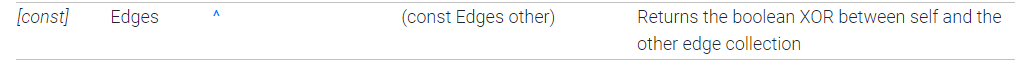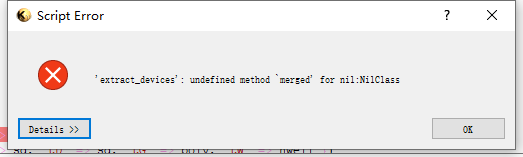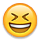# Exceptions to the results of the "xor" calculation

edited January 23

The result of the `xor`calculation for `RBA::Edges` is a `NilClass` type, which means that the result of the `xor`calculation cannot be called further because the `NilClass`does not contain the methods that would otherwise be included in `RBA::edges`.

``````  def extract_devices(layer_geometry)
sd = layer_geometry#device
s = sd
d = sd
g  = layer_geometry#device
s_region = RBA::Region.new(s)
d_region = RBA::Region.new(d)
g_region = RBA::Region.new(g)
seed = g_region
s_edges = RBA::Edges.new
(s.each_edge).each {
|n|
s_edges = s_edges + n
}
d_edges = RBA::Edges.new
(d.each_edge).each {
|n|
d_edges = d_edges + n
}
seed_edges = RBA::Edges.new
(seed.each_edge).each {
|x|
seed_edges = seed_edges + x
}
puts(s_edges^d_edges^seed_edges).merged
end
``````• You're trying to call "merged" on the result of "puts".

It has to be (note the additional brackets):

``````puts((s_edges^d_edges^seed_edges).merged)
``````

Matthias

• Thanks!!
Ah, a mistake so stupid that he bothered me for half a day